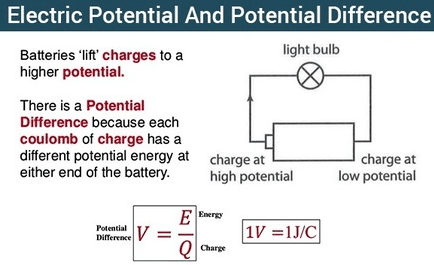Electricity & Megnetism

# Difference between electric potential and potential difference in tabular formThe basic difference between electric potential and potential difference is that electric potential at a point in an electric field is equal to the amount of work done in bringing a unit positive charge from infinity to that point. While Potential difference is the work done in carrying a unit positive charge from one point to another point while keeping the charge in equilibrium.

## Electric potential formula

If  W  is the work done in moving a unit positive charge from infinity to a certain point in the field, the electric potential V at this point would be given by:

V=W/q0

It implies that electric potential is measured relative to some reference point and like potential energy can be measured only the change in potential between two points.

It is a scalar quantity,its SI unit is volt which is equal to JC¯1. volt:”If one joule of work is done against the electric field in bringing one coulomb positive charge from infinity to a point in the electric field then the potential at that point will be one volt.” or “one joule of work done on a charge of one coulomb is equal to one volt”.

## How to find electric potential between two points?

Let us consider a positive charge q0 which is allowed to move in an electric field produced between two oppositely charged parallel plates as shown in the figure. The positive charge will move from plate B to A and will gain K.E.If it is to be moved from A to B,an external force is needed to make the charge move against the electric field and will gain P.E.Let us impose a condition that as the charge is moved from A to B, it is moved to keep electrostatic equilibrium,i.e.,it moves with uniform velocity.

This condition could be achieved by applying a force F equal and opposite to q0E at every point along its path as shown in the figure. The work done by the external force against the electric field increases the electrical potential energy of the charge that is moved. Let WAB be the work done by the force in carrying the positive charge qo from A to B while keeping the charge in equilibrium. The change in its potential energy is given by ΔU= WAB or

UB and UA =  WAB

Where  UA and Uare defined to be the potential energies at points A and B, respectively.

To describe the electric field we introduce the idea of electric potential difference. The potential difference between two points A and B in an electric field is defined as “The work is done in carrying a unit positive charge from points A to B while keeping the charge in equilibrium.

## Potential difference formula

ΔV=VB-VA=WAB/q0=ΔU/q0

Where VA and VB are defined as electric potentials at points A and B  respectively. Electric potential energy difference and the electric potential difference between points A and B  are related as

ΔU=q0ΔV=WAB

Thus the p.d between the two points can be defined as “the difference of the potential energy per unit charge.”

## Unit p.d

1volt=1joule/1coulomb

That is, a potential difference of 1 volt exists between two points if work done in moving a unit positive charge from one point to another, keeping equilibrium, is one joule. In order to give a concept of electric potential at a point in an electric field, we must have a reference to which we assign zero electric potential. this point is usually taken at infinity. Thus in equation

ΔV=VB-VA=WAB/q0

we take A to be at infinity and choose Va=0, the electric potential  at B will be

VB=WB/q0

or dropping the subscripts we have an equation

V=W/q0

which states that the electric potential at any point in an electric field is equal to work done in bringing a unit positive charge from infinity to that point keeping it in equilibrium. It is to be noted that potential at a point is still a potential difference between the potential at that point and potential at infinity. Both potential and potential differences are scalar quantities because both W and q0 are scalars. Watch the video about the electric potential and potential difference to clear your concepts visually.

Video about Difference between electric potential and potential difference
To see the difference between electric potential and potential difference watch the video given below:

Related topics